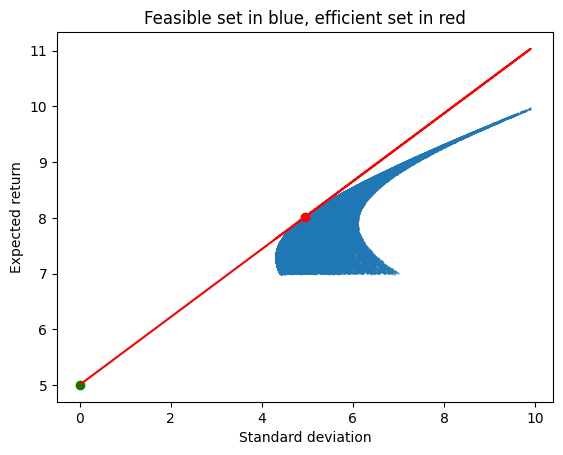# Classical portfolio theory, Julia version¶

The following is a Julia version of the core parts of our classical portfolio theory notebook.

In :
# Parameters

r=[7,10,7] # expected returns on risky assets
Omega=[49 10 -10; 10 100 10; -10 10 49] # variance-covariance matrix
rF=5 # risk-free rate
n=100000 # number of random portfolios

Out:
100000
In :
# and off we go

using Random
Random.seed!(100) # if you want the exact same numbers as me

anowone=[1/sum(row)*row for row in [rand(3) for i in 1:n]]
results=[[anowone[i]'*r,sqrt(anowone[i]'*Omega*anowone[i])] for i in 1:n]
ret=[item for item in results]
std=[item for item in results]

# now we look for the approximate market portfolio
# it is the portfolio with the highest sharpe ratio

sharpe=[(ret[i]-rF)/std[i] for i in 1:n]
imax=argmax(sharpe) # this is the index of the portfolio with the highest sharpe ratio
market=anowone[imax]
sharpemarket=sharpe[imax]
retmarket=ret[imax]
stdmarket=std[imax]

print("The approximate market portfolio is: ", market)
print("\n")
print("The market return is: ", retmarket)
print("\n")
print("The std of the market return is: ", stdmarket)
print("\n")
print("The sharpe ratio of the market return is: ", sharpemarket)

The approximate market portfolio is: [0.32983, 0.340684, 0.329486]
The market return is: 8.022051213020447
The std of the market return is: 4.957370338353463
The sharpe ratio of the market return is: 0.6096077167444764
In :
# Now we graph things

using PyPlot

scatter(std,ret,0.2)
scatter(stdmarket,retmarket,color="red")
scatter(0,rF,color="green")
plot(std, sharpemarket*std+rF*ones(n,1),color="red")
xnow=[0,stdmarket]
plot(xnow, sharpemarket*xnow+rF*ones(2,1),color="red")
# plot()

ax=gca()
ax.set_ylabel("Expected return")
ax.set_xlabel("Standard deviation")
title("Feasible set in blue, efficient set in red")Out:
PyObject Text(0.5, 1.0, 'Feasible set in blue, efficient set in red')
In :
# now we find the optimal portfolio for someone who wishes
# to maximize expected return - variance/6

using Optim
using Optim: converged, maximum, maximizer, minimizer, iterations #some extra functions

f(x)=x*rF+(1-x)*retmarket-((1-x)*stdmarket)^2/6
opt=maximize(f,-1,1) #-1 to 1 is the search interval

print("The optimal risk-free share is: ",maximizer(opt))
print("\n")
print("At the optimal, the expected return is: ",maximizer(opt)*rF+(1-maximizer(opt))*retmarket)
print("\n")
print("At the optimal, the standard deviation of the return is: ",(1-maximizer(opt))*stdmarket)

The optimal risk-free share is: 0.6310900594406986
At the optimal, the expected return is: 6.114864733362538
At the optimal, the standard deviation of the return is: 1.8288231968524196